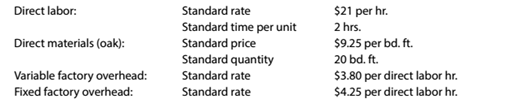Chapter 13, Problem 13.18E

Chapter
Section
Textbook Problem

Standard product cost Sorrento Furniture Company manufactures unfinished oak furniture. Sorrento uses a standard cost system. The direct labor, direct materials, and factory overhead standards for an unfinished dining room table are as follows:Determine the standard cost per dining room table.

To determine

Concept Introduction:

Standard Costing System:

Standard Costing system allows estimating the costs, preparing budgets for future periods, and analyzing the performance by comparing the budgets with actual results and find variances.

To Calculate:

The Standard Cost per unit

Explanation

The Standard Cost per unit is calculated as follows:

 Direct Labor (2 hrs * \$21) \$ 42.00 Direct Material (20 ft * \$9.25) \$ 185.00 Variable Overhead (2 hrs * \$3...

Still sussing out bartleby?

Check out a sample textbook solution.

See a sample solution

The Solution to Your Study Problems

Bartleby provides explanations to thousands of textbook problems written by our experts, many with advanced degrees!

Get Started